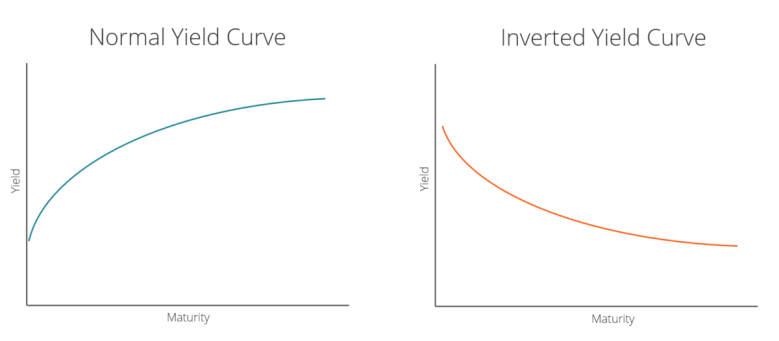An inverted yield curve is when the yields on bonds with a shorter duration are higher than the yields on bonds that have a longer duration.

It often signals a lead-up to a recession or economic slowdown.

Because yield curve inversions are rare, they typically attract attention from the financial world when it happens.

## What is an inverted yield curve?

The yield curve is a graphical representation of the relationship between the interest rate paid by an asset (usually government bonds) and the time to maturity.

The yield curve is also known as the term structure of interest rates.

The interest rate is measured on the vertical axis and time to maturity is measured on the horizontal axis.Normally, interest rates and time to maturity are positively correlated.

Under normal conditions, interest rates go up with an increase in the time to maturity. This results in a positive slope for the yield curve.

If interest rates and time to maturity are negatively correlated, then the resulting inverted yield curve will show a negative slope.

Historically, the yield curve assumes a negative slope in the lead-up to recessions and economic slowdowns.

## How to Measure an Inverted Yield Curve

The spread between a 10-year bond and a 2-year bond is often used to check for inversion of the yield curve.

If the 10-2 spread falls below 0, then the yield curve is negatively sloped on average between 24 months and 120 months (time to maturity).

## The Inverted Yield Curve Used to Forecast Future Recessions

The yield curve is often seen as the bond market’s measure of confidence in the economy.

Expectations of future interest rates shape the yield curve.

• A positive slope implies the bond market expects the economy to do well.
• A negative slope implies the bond market expects the economy to do badly.

A negatively sloped (“inverted”) yield curve implies that investors expect interest rates to be lower in the future.

This means that investment returns generally will be lower in the future.

Lower returns lead to a decrease in investments that are associated with economic stagnation and deflation.

Central banks often counter deflationary pressures in the economy by reducing short-term interest rates. Therefore, an expectation of economic slowdown is consistent with a negatively sloped yield curve.